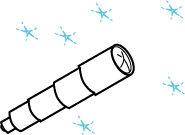creation: 09/06/99; modified 05/07/03, 08/09/17

# Fill in the BlanksInstructions:   This is an ungraded fill-in-the-blank exercise. Each question consists of a statement with one or two words left out. A button represents the missing word(s). For each question, think of the word or phrase that should fill each blank, then click on the buttons to see if you are correct. No grade is calculated for this exercise.

1.  Design of the Telescope Class. The three most important numbers describing a telescope are: the diameter of the main lens (the one in front), the focal length of the main lens, and the focal length of the eyepiece. From these values other characteristics of the telescope such as its magnification and the f-number of the main lens are calculated.

Fill in the blanks in the following design for the class:

```class

A class that models a telescope.

Constructors

public Telescope ( double ,
double ,
double   )

Methods

// calculate the magnification of the telescope
public double

// calculate the f-number of the telescope
public double
```

2.  Checking the Design. To check the design, write a small program that uses the class to see if it works well. Write a program that creates a Telescope object with a main lens that has a diameter of 3.0 inches, a focal length of 6.5 inches, and an eyepiece focal length of 0.8 inches. Write out its magnification and f-number.

```public class TelescopeTester
{

public static void main ( String[] args )
{
Telescope tele = new   ;

System.out.println( "Power: "    + tele. +
"  F-number: " + tele. );

}
}
```

3.  Skeleton of the Class. Fill in the blanks that give the over-all design of the class.

```public class Telescope
{
//

//

//

}
```

4.  Fill in Instance Variables. Fill in the data type of each instance variable.

```class Telescope
{
// Instance Variables
private   diameter;
private   mainLength;
private   eyeLength;

// Constructors

// Methods

}
```

5.  Complete the Constructor. The constructor will initialize the instance variables of the object being constructed.

```public class Telescope
{
// Instance Variables
private double diameter;
private double mainLength;
private double eyeLength;

// Constructors
public ( double , double , double   )
{
this.diameter   =     ;
this.mainLength =   ;
this.eyeLength  =    ;

}

// Methods

}
```

When an instance variable and a parameter use the same identifier, you specify the instance variable of the object by saying "this.identifier" as in the above.

6.  Complete a Method. Complete the `magnification()` method. The formula is: magnification = mainLength/eyeLength

```public class Telescope
{
// Instance Variables
private double diameter;
private double mainLength;
private double eyeLength;

// Constructors
public Telescope ( double diameter, double mainLength, double eyeLength )
{
this.diameter   = diameter ;
this.mainLength = mainLength ;
this.eyeLength  = eyeLength ;

}

// Methods
public double magnification()
{
return  /  ;
}
}
```

You don't have to use "this" in the method because it is clear that the identifiers refer to the instance variables. The `magnification()` method can not even see the parameters of the constructor.

7.  Complete the fNumber() Method. The formula to use is: fNumber = mainLength/diameter

```class Telescope
{
// Instance Variables
private double diameter;
private double mainLength;
private double eyeLength;

// Constructors
public Telescope ( double diameter, double mainLength, double eyeLength )
{
this.diameter   = diameter ;
this.mainLength = mainLength ;
this.eyeLength  = eyeLength ;
}

// Methods
public double magnification()
{
return mainLength / eyeLength ;
}

public double fNumber()
{
return  /  ;
}
}
```

8.  Compile each File.

```C:\MyFiles>javac

C:\MyFiles>javac
```

You could also just use the second command. The `javac` compiler will then do the first automatically.

9.  Run the Program. The java bytecode interpreter is started with the .class file that contains the main() method:

```C:\MyFiles>java
```

End of the Exercise. If you want to do it again, click on "Refresh" in your browser window.